# Maharashtra Board Class 11 Chemistry Important Questions Chapter 12 Chemical Equilibrium

Balbharti Maharashtra State Board 11th Chemistry Important Questions Chapter 12 Chemical Equilibrium Important Questions and Answers.

## Maharashtra State Board 11th Chemistry Important Questions Chapter 12 Chemical Equilibrium

Question 1.
Explain irreversible reaction.
Irreversible reaction:
i. Reactions which occur only in one direction, namely, from reactant to products are called irreversible reactions.
ii. They proceed in only a single direction until one of the reactants is exhausted.
iii. The direction in which an irreversible reaction occurs is indicated by an arrow (→) pointing towards the products in the chemical equation.
e.g. a. $$\mathrm{C}_{(\mathrm{s})}+\mathrm{O}_{2(\mathrm{~g})} \stackrel{\text { Burn }}{\longrightarrow} \mathrm{CO}_{2(\mathrm{~g})}$$
b. $$2 \mathrm{KClO}_{3(\mathrm{~s})} \stackrel{\Delta}{\longrightarrow} 2 \mathrm{KCl}_{(\mathrm{s})}+3 \mathrm{O}_{2(\mathrm{~g})}$$

Question 2.
What is a closed system?
A system in which there is no exchange of matter with the surroundings is called a closed system.

Question 3.
What is an open system?
A system in which exchange of both matter and heat occurs with the surroundings is called an open system.

Question 4.
Why was calcium oxide used in theatre lighting?
Calcium oxide (CaO) on strong heating glows with a bright white light. Hence, CaO was used in theatre lighting, which gave rise to the phrase ‘in the limelight’.Question 5.
Explain liquid-vapour equilibrium with an example.
Liquid-vapour equilibrium:
i. Consider reversible physical process of evaporation of liquid water into water vapour in a closed vessel. Initially, there is practically no water vapour in the vessel.

ii. When the liquid evaporates in the closed container, the liquid molecules escape from the liquid surface into vapour phase building up vapour pressure. They also condense back into liquid state because the container is closed.

iii. In the beginning the rate of evaporation is high and the rate of condensation is low. But with time, as more and more vapour is formed, the rate of evaporation goes down and the rate of condensation increases. Eventually the two rates become equal. This gives rise to a constant vapour pressure. This state is known as an ‘equilibrium state’.
In this state, the rate of evaporation is equal to the rate of condensation.
It may be represented as: H2O(l) ⇌ H2O(Vapour)

iv. At equilibrium, the pressure exerted by the gaseous water molecules at a given temperature remains constant, known as the equilibrium vapour pressure of water (or saturated vapour pressure of water or aqueous tension). The saturated vapour pressure increases with increase of temperature.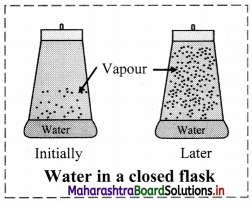[Note: The saturated vapour pressure of water at 100 °C is 1 atm (1.013 bar). Hence, water boils at 100 °C when pressure is 1 atm.]

Question 6.
What is meant by the term ‘normal boiling point’ of a liquid?
For any pure liquid at 1 atm pressure, the temperature at which its saturated vapour pressure equals to atmospheric pressure is called the normal boiling point of that liquid.
e.g. The boiling point of ethyl alcohol is 78 °C i.e., the saturated vapour pressure of ethyl alcohol at 78 °C is 1 atm (1.013 bar).

Question 7.
Give an example of solid-liquid equilibrium.
A mixture of ice and water in a perfectly insulated thermos flask at 273 K is an example of solid-liquid equilibrium.
H2O(s) ⇌ H2O(l)

Question 8.
Identify the type of equilibrium in the following physical processes:
i. Camphor(s) ⇌ Camphor(g)
ii. Ammonium chloride(s) ⇌ Ammonium chloride(g)
iii. Carbon dioxide gas ⇌ Dry ice
iv. Water ⇌ Ice
i. Solid – vapour equilibrium
ii. Solid – vapour equilibrium Solid
iii. Solid – vapour equilibrium
iv. Solid – liquid equilibriumQuestion 9.
Name two substances that undergoes sublimation.
Camphor, ammonium chloride.

Question 10.
Write a short note on chemical equilibrium.
Chemical equilibrium:

• If a reaction takes place in a closed system so that the products and reactants cannot escape, we often find that reaction does not give a 100% yield of products. Instead some reactants remain after the concentrations stop changing.
• When there is no further change in concentration of reactant and product, the chemical reaction has attained equilibrium, with the rates of forward and reverse reactions being equal.
• Chemical equilibrium at a given temperature is characterized by constancy of measurable properties such as pressure, concentration, density, etc.
• Chemical equilibrium can be approached from either side of the chemical reaction.

Question 11.
Explain the law of mass action and give its mathematical representation.
Statement: The law of mass action states that the rate of a chemical reaction at each instant is proportional to the product of concentrations of all the reactants.
Explanation: A rate equation can be written for a reaction by applying the law of mass action as follows: Consider a reaction, A + B → C
Here A and B are the reactants and C is the product. The concentrations of chemical species are expressed in mol L-1 and denoted by putting the formula in square brackets. On applying the law of mass action to this
reaction, a proportionality expression can be written as: Rate ∝ [A] [B]
This proportionality expression is transformed into an equation by introducing a proportionality constant, k, as follows:
Rate = k [A] [B]
This equation is called the rate equation and the proportionality constant, k, is called the rate constant of the reaction.

Question 12.
Write the rate equation for the following reactions:
i. C + O2 → CO2
ii. 2KClO3 → 2KCl + 3O2
The rate equation is written by applying the law of mass action.
i. The reactants are C and O2
Rate ∝ [C] [O2]
∴ Rate = k [C] [O2]
ii. The reactant is KClO3 and its 2 molecules appear in the balanced equation.
∴ Rate ∝ [KClO3]2
∴ Rate = k [KClO3]2Question 13.
Derive the expression of equilibrium constant, KC for the reaction:
A + B ⇌ C + D
Consider a hypothetical reversible reaction A + B ⇌ C + D.
Two reactions, namely, forward and reverse reactions occur simultaneously in a reversible chemical reaction. The rate equations for the forward and reverse reactions are:
Rateforward ∝ [A][B]
∴ Rateforward = kf [A] [B] …… (1)
∴ Ratereverse ∝ [C] [D]
∴ Ratereverse = kr [C] [D] …. (2)
At equilibrium, the rates of forward and reverse reactions are equal. Thus,
Rateforward = Ratereverse
∴ kf [A] [B] = kr [C] [D]
∴ $$\frac{\mathrm{k}_{\mathrm{f}}}{\mathrm{k}_{\mathrm{r}}}=\mathrm{K}_{\mathrm{C}}=\frac{[\mathrm{C}][\mathrm{D}]}{[\mathrm{A}][\mathrm{B}]}$$ …….. (3)
KC is called the equilibrium constant.

Question 14.
Show that the equilibrium constant of the reverse chemical reaction (KC) is the reciprocal of the equilibrium constant (KC).
Consider a reversible chemical reaction:
aA + bB ⇌ cC + dD
The equilibrium constant, KC = $$\frac{[C]^{c}[D]^{d}}{[A]^{a}[B]^{b}}$$
Consider the reverse reaction:
cC + dD ⇌ aA + bB.
The equilibrium constant, KC is:
KC = $$\frac{[\mathrm{A}]^{a}[\mathrm{~B}]^{\mathrm{b}}}{[\mathrm{C}]^{\mathrm{c}}[\mathrm{D}]^{\mathrm{d}}}=\frac{1}{\mathrm{~K}_{\mathrm{C}}}$$
Thus, equilibrium constant of the reverse chemical reaction (KC) is the reciprocal of the equilibrium constant KC.

Question 15.
Write equilibrium constant expressions for both forward and reverse reaction for the synthesis of ammonia by the Haber process.
Synthesis of ammonia by Haber process:
N2(g) + 3H2(g) ⇌ 2NH3(g)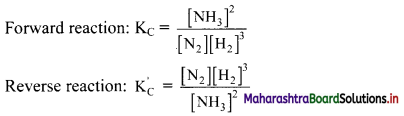Question 16.
How are the equilibrium constants of the following pair of equilibrium reactions related?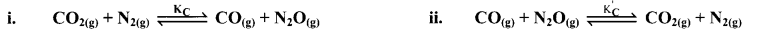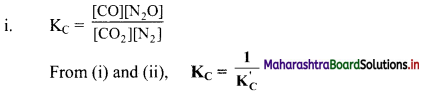ii. KC = $$\frac{\left[\mathrm{CO}_{2}\right]\left[\mathrm{N}_{2}\right]}{[\mathrm{CO}]\left[\mathrm{N}_{2} \mathrm{O}\right]}$$

Question 17.
Write KP expression for the reaction:
aA(g) + bB(g) ⇌ cC(g) + dD(g)
For the given reaction,
KP = $$\frac{\left(P_{c}\right)^{c}\left(P_{D}\right)^{d}}{\left(P_{A}\right)^{a}\left(P_{B}\right)^{b}}$$

Question 18.
N2(g) + 3H2(g) ⇌ 2NH3(g)
Write expressions for KP and substitute expressions for PN2, PH2 and PNH3 using ideal gas equation.
For the given reaction, KP = $$\frac{\left(P_{N H_{3}}\right)^{2}}{\left(P_{N_{2}}\right)\left(P_{H_{2}}\right)^{3}}$$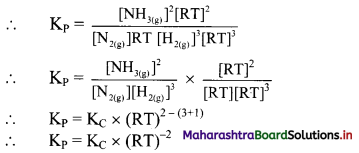[Note: The above question is modified to apply appropriate textual context, i. e., to indicate that students need to use ideal gas equation to derive expressions for PN2, PH2 and PNH3]Question 19.
For a chemical equilibrium reaction
H2(g) + I2(g) ⇌ 2HI(g),
write an expression for KP (and relate it to KC).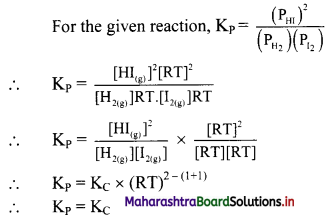Question 20.
Write the relationship between KC and KP for the following equilibria: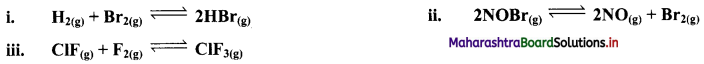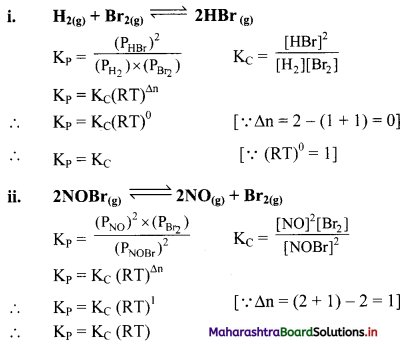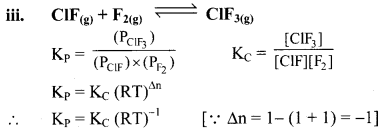Question 21.
Write the expressions for KC and KP and the relationship between them for the equilibrium reaction,
2A(g) + B(g) ⇌ 3C(g) + 2D(g)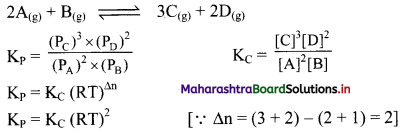Question 22.
Explain in short homogeneous equilibrium and heterogeneous equilibrium.
i. In a homogeneous equilibrium, the reactants and products are in the same phase.
e.g. Dissociation of HI:
2HI(g) ⇌ H2(g) + I2(g)
ii. In a heterogeneous equilibrium, the reactants and products exist in different phases, e.g. Formation of NH4Cl:
NH3(g) + HCl(g) ⇌ NH4Cl(s)Question 23.
The unit of KC is different for different reactions. Explain this statement with suitable examples.
Unit of equilibrium constant:
i. The unit of equilibrium constant depends upon the expression of KC which is different for different equilibria. Therefore, the unit of KC is also different for different reactions.
ii. Consider the following equilibrium reaction: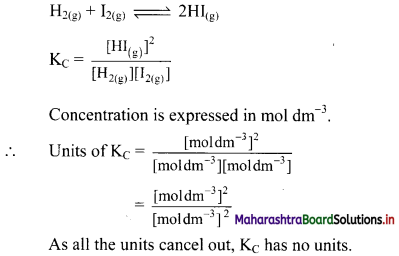iii. Consider the following equilibrium reaction: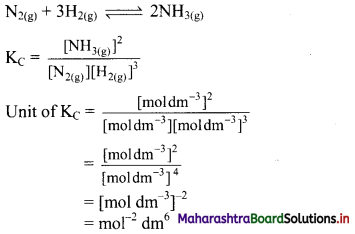Question 24.
Write the equilibrium constant expression for the decomposition of baking soda. Deduce the unit of KC from the above expression.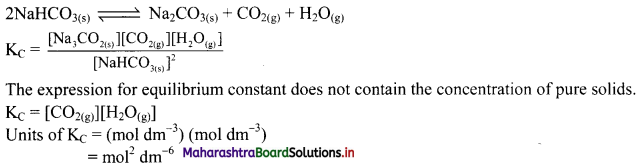[Note: Considering gaseous reactants and products, in this reaction, Δn = 2 – 0 = 2
∴ Units of KC = (mol dm-3)Δn
= (mol dm-3)2
= mol2 dm-6
Thus, the units of the above reaction is mol2 dm-6.]

Question 25.
What are the characteristics of equilibrium constant?
Characteristics of equilibrium constant:

• The value of equilibrium constant is independent of initial concentrations of either the reactants or products.
• Equilibrium constant is temperature dependent. Hence, KC and KP change with change in temperature.
• Equilibrium constant has a characteristic value for a particular reversible reaction represented by a balanced equation at a given temperature.
• Higher value of KC or KP means more concentration of products is formed and the equilibrium point is more towards right hand side and vice versa.

Question 26.
Explain how equilibrium constant helps in predicting the direction of the reaction.
Prediction of the direction of the reaction:
i. For the reaction, aA + bB ⇌ cC + dD,
The equilibrium constant (KC) is given as:
KC = $$\frac{[C]^{c}[D]^{d}}{[A]^{a}[B]^{b}}$$
where, all the concentrations are equilibrium concentrations.
ii. When the reaction is not necessarily at equilibrium, the concentration ratio is called QC i.e.,
QC = $$\frac{[C]^{c}[D]^{d}}{[A]^{a}[B]^{b}}$$
iii. By comparing QC with KC for a reaction under given conditions, we can decide whether the forward or the reverse reaction should occur to establish the equilibrium.
a. QC < KC: The reaction will proceed from left to right, in forward direction, generating more product to attain the equilibrium.
b. QC > KC: The reaction will proceed from right to left, removing product to attain the equilibrium.
c. QC = KC: The reaction is at equilibrium and no net reaction occurs.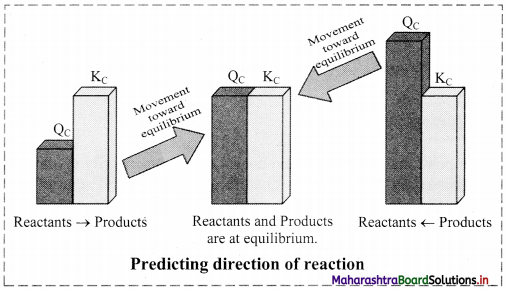[Note: The prediction of the direction of the reaction on the basis of QC and KC values makes no comment on the time required for attaining the equilibrium.]Question 27.
Explain how KC can be used to know the extent of the reaction?
Extent of the reaction: The equilibrium constant expression indicates that the magnitude of KC is:
i. directly proportional to the concentrations of the products.
ii. inversely proportional to the concentrations of the reactants.
a. Value of KC is very high (KC > 103):
At equilibrium, there is a high proportion of products compared to reactants.
Forward reaction is favoured.
Reaction is in favour of products and nearly goes to completion.

b. Value of KC is very low (KC < 10-3):
At equilibrium, only a small fraction of the reactants is converted into products.
Reverse reaction is favoured.
Reaction hardly proceeds towards the products.

c. Value of KC is in the range of 10-3 to 103:
Appreciable concentrations of both reactants and products are present at equilibrium.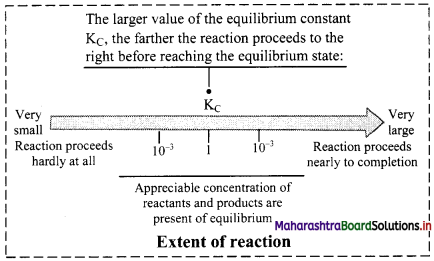Question 28.
For the following reactions, write KC expressions and predict direction of the reactions based on the magnitude of their equilibrium constants.
i. 2H2(g) + O2(g) ⇌ 2H2O(g), KC = 2.4 × 1047 at 500 K
ii. 2H2O(g) ⇌ 2H2(g) + O2(g), KC = 4.2 × 10-48 at 500 K
i. a. KC expression:
KC = $$\frac{\left[\mathrm{H}_{2} \mathrm{O}_{(\mathrm{g})}\right]^{2}}{\left[\left[\mathrm{H}_{2(\mathrm{~g})}\right]\right]^{2}\left[\mathrm{O}_{2(\mathrm{~g})}\right]}$$
b. For the reaction, KC = 2.4 × 1047 at 500 K
If the value of KC >>> 103, forward reaction is favoured.
Hence, the given reaction will proceed in the forward direction and will nearly go to completion.

ii. a. KC expression:
KC = $$\frac{\left[\mathrm{H}_{2(\mathrm{~g})}\right]^{2}\left[\mathrm{O}_{2(\mathrm{~g})}\right]}{\left[\mathrm{H}_{2} \mathrm{O}_{(\mathrm{g})}\right]^{2}}$$
b. For the reaction, KC = 4.2 × 10-48 at 500 K
If the value of KC <<< 10-3, reverse reaction is favoured.
Hence, the given reaction will proceed in the backward direction and will nearly go to completion.

Question 29.
Describe how equilibrium constant can be used to calculate the composition of an equilibrium mixture.
An equilibrium constant can be used to calculate the composition of an equilibrium mixture.
Consider an equilibrium reaction, A(aq) + B(aq) ⇌ C(aq) + D(aq)
The equilibrium constant is 4.0 at a certain temperature.
Let the initial amount of A and B be 2.0 mol in ‘V’ litres. Let x mol be the equilibrium amount of C.
Hence, we can construct a table as shown below: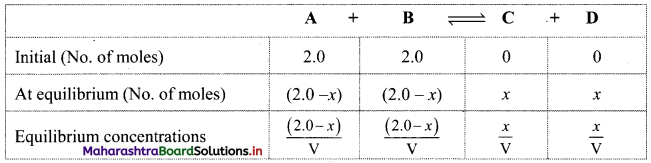The expression for equilibrium constant can be written as: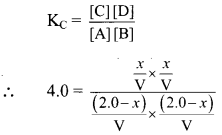Substituting the value of equilibrium concentration, we get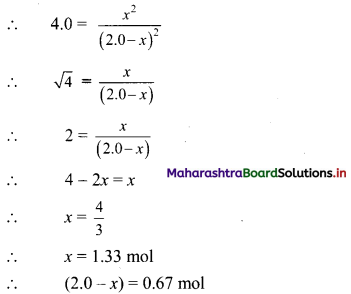Therefore, equilibrium concentrations are 0.67 mol of A, 0.67 mol of B, 1.33 mol of C and 1.33 mol of D in V litres.

Question 30.
Explain the link between chemical equilibrium and chemical kinetics:
Equilibrium constant (KC) is related to rate or velocity constants of forward reaction (kf) and reverse reaction (kr) as:
KC = $$\frac{\mathrm{k}_{\mathrm{f}}}{\mathrm{k}_{\mathrm{r}}}$$
This equation can be used to determine the composition of the reaction mixture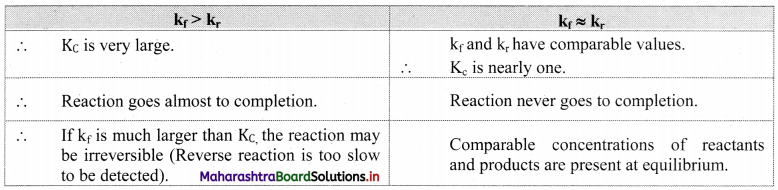[Note: The equilibrium refers to the relative amounts of reactants and products and thus a shift in equilibrium in a particular direction will imply the reaction in that direction will be favoured.]Question 31.
Equal concentrations of hydrogen and iodine are mixed together in a closed container at 700 K and allowed to come to equilibrium. If the concentration of HI at equilibrium is 0.85 mol dm-3, what are the equilibrium concentrations of H2 and I2 if KC = 54 at this temperature?
Solution:
Given: [HI(g)] = 0.85 mol dm-3
KC = 54 at 700 K
Equilibrium concentrations of H2 and I2
Formula: KC = $$\frac{[\mathrm{C}]^{\mathrm{c}}[\mathrm{D}]^{\mathrm{d}}}{[\mathrm{A}]^{\mathrm{a}}[\mathrm{B}]^{\mathrm{b}}}$$
Balanced chemical reaction: 2HI(g)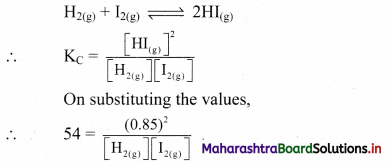Equilibrium concentration of I2(g) = Equilibrium concentration of H2(g)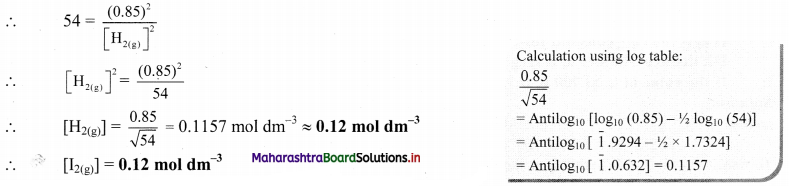Ans: Equilibrium concentrations of H2 and I2 are equal to 0.12 mol dm-3.

Question 32.
Calculate Kc at 500 K for the reaction,
2HI(g) ⇌ H2(g) + I2(g) if the equilibrium concentrations are [HI] = 0.5 M, [H2] = 0.08 M and [I2] = 0.062 M.
Solution:
Given: T = 500 K,
At equilibrium, [HI] = 0.5 M, [H2] = 0.08 M, [I2] = 0.062 M.
To find: Equilibrium constant KC
Formula: KC = $$\frac{[C]^{c}[D]^{d}}{[A]^{a}[B]^{b}}$$
Calculation: The above equilibrium reaction is given as 2HI(g) ⇌ H2(g) + I2(g)
The expression of KC is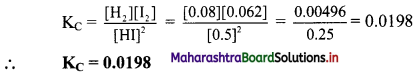Ans: KC at 500 K for the given reaction is 0.0198.

Question 33.
Calculate KC and KP for the reaction at 295 K, N2O4 ⇌ 2NO2(g) if the equilibrium concentrations are [N2O4] = 0.75 M and [NO2] = 0.062 M, R = 0.08206 L atm K-1 mol-1.
Solution:
Given: R = 0.08206 L atm K-1 mol-1, T = 295 K
At equilibrium , [N2O4] = 0.75 M, [NO2] = 0.062 M
To find: Equilibrium constants, KP and KC
Formulae: i. KC = $$\frac{[\mathrm{C}]^{\mathrm{c}}[\mathrm{D}]^{\mathrm{d}}}{[\mathrm{A}]^{\mathrm{a}}[\mathrm{B}]^{\mathrm{b}}}$$
ii. KP = KC (RT)Δn
Calculation : The equilibrium reaction is given as N2O4(g) ⇌ 2NO2(g)
The expression of KC is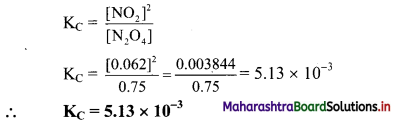KP is related to KC by expression: KP = KC (RT)Δn
where, Δn = numbers of moles of gaseous products – number of moles of gaseous reactants
= 2 – 1 = 1
∴ KP = KC(RT)1
∴ KP = 5.13 × 10-3 × 0.08206 × 295
∴ KP= 123.9 × 10-3 = 0.124
Ans: KC and KP for the reaction at 295 K are 5.13 × 10-3 and 0.124 respectively.

Question 34.
The equilibrium constant KC for the reaction of hydrogen with iodine is 54.0 at 700 K.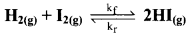KC = 54.0 at 700 K
If kf is the rate constant for the formation of HI and kr is the rate constant for the decomposition of HI, deduce whether kr is larger or smaller than kr.
ii. If the value of kr at 700 K is 1.16 × 10-3, what is the value of kf ?
Solution:
Given: i. KC = 54.0 at 700 K
ii. kr = 1.16 × 10-3 at 700 K
To find: i. Whether kf is larger or smaller than kr.
ii. Value of kf.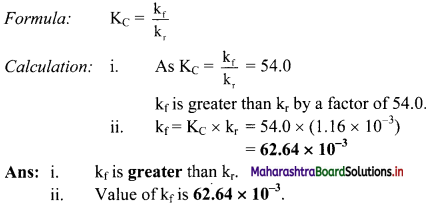Question 35.
Given the equilibrium reaction, H2O(g) + CH4(g) ⇌ CO(g) + 3H2(g)
Using Le Chatelier’s principle, predict how concentration of CO will change when the equilibrium is disturbed by
iii. removing H2O
iv. removing H2
i. Adding CH4: Adding CH4 will favour the forward reaction and the yield of CO and H2 will increase.
ii. Adding H2: Adding H2 will favour the reverse reaction and the yield of CO and H2 will decrease.
iii. Removing H2O: Removing H2O will favour the reverse reaction and the yield of CO and H2 will decrease.
iv. Removing H2: Removing H2 will favour the forward reaction and the yield of CO and H2 will increase.Question 36.
By using Le Chatelier’s principle, explain the effect of change in pressure (due to volume change) on the composition of equilibrium mixture.
Change in pressure:
i. The change in pressure has no effect on the concentrations of solids and liquids. However, it appreciably affects the concentrations of gases.
From the ideal gas equation, PV = nRT or P = $$\frac{\mathrm{n}}{\mathrm{V}}$$RT
∴ P ∝ $$\frac{\mathrm{n}}{\mathrm{V}}$$
where, the ratio n/V is an expression for the concentration of the gas in mol dm-3.
ii. According to Le Chatelier’s principle at constant temperature, when pressure is increased, the equilibrium will shift in a direction in which the number of molecules decreases and when the pressure is decreased the equilibrium will shift in a direction in which the number of molecules increases.

[Note: For a reaction in which decrease in volume takes place, the reaction will be favoured by increasing pressure and for a reaction in which increase in volume takes place, the reaction will be favoured with lowering pressure, temperature being constant.]

Question 37.
An equilibrium mixture of dinitrogen tetroxide (colourless gas) and nitrogen dioxide (brown gas) is set up in a sealed flask at a particular temperature. Observe the effect of change of pressure on the gaseous equilibrium and complete the following table: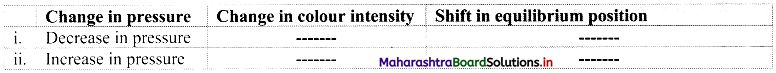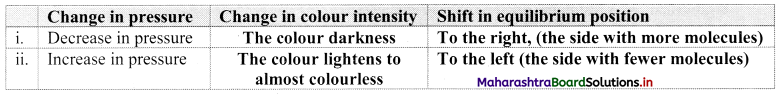Question 38.
By using Le Chatelier’s principle, explain the effect of change in pressure for the following equilibrium:
H2(g) + I2(g) ⇌ 2HI(g)
As there is the same number of molecules of gas on both sides, change of pressure has no effect on the equilibrium.

Question 39.
Explain the effect of change in pressure due to volume change of the following equilibria:
i. 2NO(g) + Cl2(g) ⇌ 2NOCl(g)
ii. 2NO(g) ⇌ N2(g) + O2(g)
i. 2NO(g) + Cl2(g) ⇌ 2NOCl(g)
In the forward reaction, the number of molecules decreases (3 to 2) and in the reverse reaction the number of molecules increases (2 to 3).
a. Effect of increase in pressure: According to Le Chatelier’s principle, when pressure is increased the forward reaction is favoured as the number of molecules decreases. Thus, when the pressure of the equilibrium system is increased at constant temperature by reducing the volume, the yield of NOCl increases.
b. Effect of decrease in pressure: When the pressure is decreased the equilibrium will shift from right to left. Therefore, the yield of NOCl will decrease.

ii. 2NO(g) ⇌ N2(g) + O2(g)
As both reactants and products have equal numbers of moles (or molecules), there is no effect of change in pressure (due to volume change) on the composition of the equilibrium mixture.

Question 40.
Explain the effect of change in temperature on the value of KC.

• The value of equilibrium constant is unaffected if temperature remains constant.
• However, a change in temperature alters the value of equilibrium constant.
• In a reversible reaction, one of the reactions is exothermic (heat is released) and the other is endothermic (heat is absorbed).
• The value of equilibrium constant for an exothermic reaction decreases with increase in the temperature and that of endothermic reaction increases with the increase in temperature.

Question 41.
Explain the effect of change in temperature on the following equilibria:
CO(g) + 2H2(g) ⇌ CH3OH(g) ; ΔH = – 90 kJ
i. The forward reaction is exothermic and reverse reaction is endothermic. According to Le Chatelier’s principle, when the temperature of the equilibrium mixture increases, the equilibrium shifts from right to left in endothermic direction. Therefore, the yield of CH3OH decreases at high temperature.

ii. When the temperature decreases, the forward exothermic reaction is favoured. Therefore, the yield of CH3OH increases at low temperature.
Thus, the decomposition of CH3OH into CO and H2 is favoured with increase in temperature, whereas formation of CH3OH is favoured with decrease in temperature.Question 42.
By using Le Chatelier’s principle, explain the effect of addition of a catalyst on the composition of equilibrium mixture.

• When a catalyst is added to the equilibrium mixture, the rates of forward and reverse reactions increases to the same extent. Hence, the position of equilibrium remains unaffected.
• A catalyst does not change the composition of equilibrium mixture. The equilibrium concentrations of reactants and products remain same and catalyst does not shift the equilibrium in favour of either reactants or products.
• The value of equilibrium constant is also not affected by the presence of a catalyst.

[Note: A catalyst does not appear in the balanced chemical equation and in the equilibrium constant expression.]

Question 43.
Consider an esterification reaction: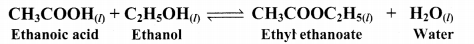What will happen if H+ ions are added to the reaction mixture?
H+ ions act as catalyst in the esterification reaction. Hence, the addition of H+ ions reduces the time for the completion of reaction.

Question 44.
Complete the following table that shows the shifts in the equilibrium position for the reaction:
N2O4(g) + Heat ⇌ 2NO2(g)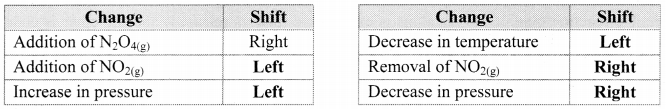Question 45.
Summarize effects of following four factors on the position of equilibrium and value of KC:
i. Concentration
ii. Pressure
iii. Temperature
iv. Catalyst

 Effect of Position of equilibrium Value of KC Concentration Changes No change Pressure Changes if reaction involves change in number of gas molecules No change Temperature Change Change Catalyst No change No change

Question 46.
State TRUE or FALSE. Correct the false statement.
i. The value of equilibrium constant depends on temperature.
ii. If QC < KCC, the reaction will proceed from right to left consuming more product to attain equilibrium.
iii. Any change in the pressure of a gaseous reaction mixture at equilibrium, changes the value of KC.
iv. In a reversible reaction, the reverse reaction has an energy change that is equal and opposite to that of the forward reaction.
i. True
ii. False
If QC > KC the reaction will proceed from right to left consuming more product to attain equilibrium.
iii. False
Any change in the pressure of a gaseous reaction mixture at equilibrium, does not change the value of KC.
iv. TrueQuestion 47.
Draw the flowchart showing the manufacture of NH3 by Haber process.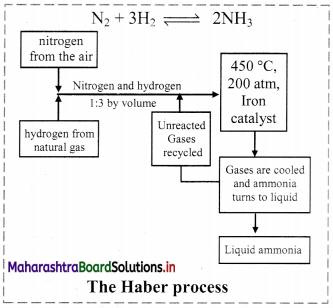Question 48.
Explain in short: The Haber process.
Haber process:

• The Haber process is the process of synthesis of ammonia gas by reacting together hydrogen gas and nitrogen gas in a particular stoichiometric ratio by volumes and at selected optimum temperature and pressure.
• The chemical reaction is: $$\mathrm{N}_{2(\mathrm{~g})}+3 \mathrm{H}_{2(\mathrm{~g})} \stackrel{\text { Catalyst }}{\rightleftharpoons} 2 \mathrm{NH}_{3(\mathrm{~g})}+\text { Heat }$$
The reaction proceeds with a decrease in number of moles (Δn = -2) and the forward reaction is exothermic.
• Iron (containing a small quantity of molybdenum) is used as catalyst.
• The optimum temperature is about 773 K and the optimum pressure is about 250 atm.

Question 49.
Consider the reaction P(g) + Q(g) ⇌ PQ(g). Diagram ‘X’ represents the reaction at equilibrium.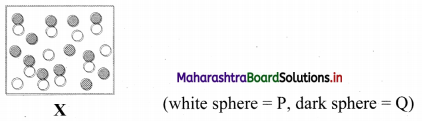i. If each molecule (sphere) represents a partial pressure of 1 atm, calculate the value of KP.
ii. Predict the change in equilibrium, when the volume is increased by 50 percentage.
i. For the given equilibrium mixture:

 Chemical species P Q PQ Partial pressure 4 6 7

KP = $$\frac{\mathrm{p}_{\mathrm{PQ}}}{\mathrm{p}_{\mathrm{p}} \times \mathrm{p}_{\mathrm{Q}}}=\frac{7}{4 \times 6}$$ = 0.29
ii. Increasing the volume will shift the equilibrium position to the side with higher number of gaseous moles. In the given reaction, the equilibrium will shift to the left (toward reactant) resulting in an increase in the concentration of P and Q accompanied by a corresponding decrease in concentration of PQ.Multiple Choice Questions

1. Which of the following is expression of KC for
2NH3(g) ⇌ N2(g) + 3H2(g)?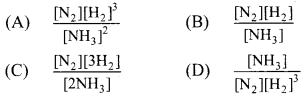(A) $$\frac{\left[\mathrm{N}_{2}\right]\left[\mathrm{H}_{2}\right]^{3}}{\left[\mathrm{NH}_{3}\right]^{2}}$$

2. For the system 3A + 2B ⇌ C, the expression for equilibrium constant is …………..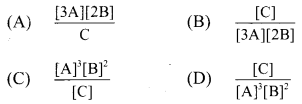(D) $$\frac{[\mathrm{C}]}{[\mathrm{A}]^{3}[\mathrm{~B}]^{2}}$$

3. For the reaction C(s) + CO2(g) ⇌ 2CO(g) the partial pressure of CO2 and CO are 4 and 8 atm, respectively, then KP for the reaction is ……………
(A) 16 atm
(B) 2 atm
(C) 5 atm
(D) 4 atm
(A) 16 atm

4. The equilibrium constant value for the reaction:
2H2(g) + O2(g) ⇌ 2H2O(g) is 2.4 × 1047 at 500 K. What is the value of equilibrium constant for the reaction:
2H2O(g) ⇌ 2H2(g) + O2(g) ?
(A) 0.41 × 10-46
(B) 0.41 × 1047
(C) 0.41 × 10-48
(D) 0.41 × 10-47
(D) 0.41 × 10-475. For the reaction CO(g) + Cl2(g) ⇌ COCl2(g), KP/KC is equal to ……………
(A) $$\frac{1}{\mathrm{RT}}$$
(B) RT
(C) $$\sqrt{\mathrm{RT}}$$
(D) 1.0
(A) $$\frac{1}{\mathrm{RT}}$$

6. For which of the following reaction, KP = KC?
(A) PCl5(g) ⇌ PCl3(g) + Cl2(g)
(B) N2(g) + 3H2(g) ⇌ 2NH3(g)
(C) H2(g) + I2(g) ⇌ 2HI(g)
(D) 2NO2(g) ⇌ N2O4(g)
(C) H2(g) + I2(g) ⇌ 2HI(g)

7. For the equilibrium reaction
2NO2(g) ⇌ N2O4(g) + 60.0 kJ, the increase in temperature ……………..
(A) favours the formation of N2O4
(B) favours the decomposition of N2O4
(C) does not affect the equilibrium
(D) stops the reaction
(B) favours the decomposition of N2O4

8. The following reaction occurs in the blast furnace where iron ore is reduced to iron metal:
3Fe2O3(s) + 3CO(g) ⇌ 2Fe(l) + 3CO2(g)
Using the Le Chatelier’s principle, predict which one of the following will NOT disturb the equilibrium?
(A) Removal of CO
(B) Removal of CO29. The reaction A + B ⇌ C + D + heat, has reached equilibrium. The reaction may be made to proceed forward by
(C) decreasing the temperature
(D) increasing the temperature
(C) decreasing the temperature

10. Identify the CORRECT statement.
(A) Catalyst lowers activation energy for the forward and reverse reactions by exactly the same amount.
(B) The value of equilibrium constant decreases in presence of a catalyst.
(C) Catalyst affect the position of the equilibrium.
(D) Catalyst changes the equilibrium composition of a reaction mixture.
(A) Catalyst lowers activation energy for the forward and reverse reactions by exactly the same amount.

11. The equilibrium constant for the reaction:
N2(g) + O2(g) ⇌ 2NO(g) is 4 × 10-4 at 2000 K. In presence of a catalyst, the equilibrium is attained ten times faster. Therefore, the equilibrium constant in presence of catalyst of 2000 K is …………..
(A) 40 × 10-4
(B) 4 × 10-2
(C) 4 × 10-3
(D) 4 × 10-4